# How To Auto Sort Data By Any Column Using VBA in Microsoft Excel 2010

In this article, you will learn how to automatically sort data by a particular column.

Let us take an example:

Following is a snapshot of the data we have. Refer below snapshot: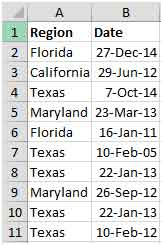We want a code that will automatically sort the data on column A as we type.

Click on Developer tab

From Code group, select Visual Basic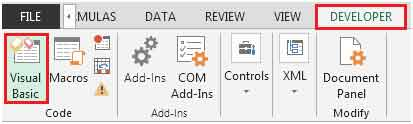Enter the following code on the sheet you are working. (sheet1 in our example)

Private Sub Worksheet_Change(ByVal Target As Range)

On Error Resume Next

If Not Intersect(Target, Range("A:A")) Is Nothing Then

Range("A1").Sort Key1:=Range("A2"), _

Order1:=xlAscending, Header:=xlYes, _

OrderCustom:=1, MatchCase:=False, _

Orientation:=xlTopToBottom

End If

End Sub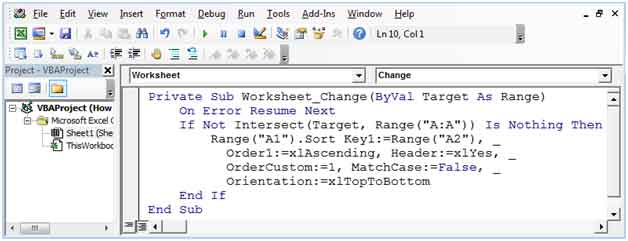Following is the result of the above code.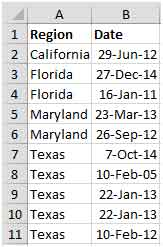The above code will activate whenever there is change in column A, if you want to activate the macro when there is any change in column B, then you have to make slight adjustment

Private Sub Worksheet_Change(ByVal Target As Range)

On Error Resume Next

If Not Intersect(Target, Range("B:B")) Is Nothing Then

Range("B1").Sort Key1:=Range("B2"), _

Order1:=xlAscending, Header:=xlYes, _

OrderCustom:=1, MatchCase:=False, _

Orientation:=xlTopToBottom

End If

End Sub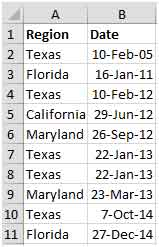In this way, you can easily sort the data by any specific column. The code will get triggered & sort the data automatically as you type information in column A (in first example) & column B (in second example). This will save a lot of time if we have to sort the data on a regular basis.

## Comments

1.Hi, from "range (C1)" line to end of "orientation" line has gone red. I have typed as directed above changing only what column to use (in my case to C). Please can you advise as I'm sure this is an error as worksheet data not changing at all when values change.

2.I copied and pasted and keep getting a syntax error

Terms and Conditions of use

The applications/code on this site are distributed as is and without warranties or liability. In no event shall the owner of the copyrights, or the authors of the applications/code be liable for any loss of profit, any problems or any damage resulting from the use or evaluation of the applications/code.